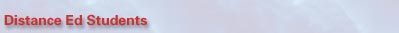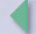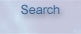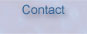# 1-2: Evolution of the Earth's Atmosphere

1-2: En Español 1-2: Em Português
© Eugene S. Takle and Richard C. Seagrave, 2002, 2005, 2006

GOAL:

To use some basic physics to help us understand the long-term characteristics of the atmosphere: why the atmosphere is what it is, how it got that way, and what is necessary to make significant changes in its structure and composition.

Escape Velocity

Escape Velocity

We begin by looking at the solar system, the physical properties of planets, and their relationship to the sun. Figure 1 gives various characteristics of bodies in our solar system that have atmospheres, listed in order of their distance from the sun (except for Titan, which is a satellite of Saturn). The first column gives the size of the body as indicated by its radius, Rp. Size of the planet determines its gravitational acceleration, as can be seen by the correlation between radius and go in the second column. Gravity, in turn, controls the escape velocity. Ve, given in column 3, which is the minimum speed that molecules must move if they are able to escape from the gravitational pull of the body. Earth, for example, being the fourth smallest planet on this list, has the fourth smallest escape velocity of 11.2 km/s or about 7 miles per second. If we wanted to launch a spacecraft to be completely free of the earth's gravitational field, it would have to have a speed of 7 miles per second. Just putting a spacecraft in orbit, of course, is a quite different problem, because the orbital parameters are determined by the balance of gravitational force and the motion of the spacecraft as will be shown in the unit on satellites.

The escape velocity can be calculated from a balance of gravitational energy at the planet surface and kinetic energy:

mgRp = 1/2 mVe2

--> Ve = (2gRp)1/2

Where m is the mass of a molecule, Rp is the radius of the planet, and Ve is escape velocity.

This shows that the escape velocity does not depend on the mass of the particle trying to escape, so the escape velocity is the same for a space ship as for a hydrogen molecule.

Albedo

Albedo

Albedo (A), given in column 5, is the fraction of energy incident on the planet that is reflected back to space. On the other hand, 1-A is the fraction of energy that is absorbed by the planet and its atmosphere. A perfectly absorbing body has an albedo of zero, and a perfectly reflecting body has an albedo of 1.0. The earth has an albedo of 0.29, which is about average for most planets. Albedo and distance from the sun are the factors determining the effective temperature of the body. Albedo is determined by the combined effects of reflection from the planet's surface and the reflecting and absorbing properties of its atmosphere, as determined by its composition.

Probable Velocity

Probable Velocity

The effective temperature of the body gives an approximation of the temperature of the gaseous constituents at the "outer edge" of its atmosphere. This temperature determines the most probable velocity of each constituent in this region, as given by the following equation:

VM = (2kT/MmH)1/2

where

VM = most probable velocity for molecule of weight M

k = Boltzmann's constant (1.38 x 10-23 J deg-1)

T = effective temperature

M = molecular weight of a particular gas species

mH = mass of the hydrogen atom ( 1.67 x 10-27kg)

Note that more massive molecules, such as CO2 with molecular weight 44, have much lower probable velocity than hydrogen with molecular weight 1 or helium with molecular weight 4. This means that for a given planet with a given gravitational acceleration and escape velocity, the lighter molecules are more likely to exceed the escape velocity and leave the planet's atmosphere. The seventh column in the table gives the most probable velocity of hydrogen (VH) for temperature corresponding to Te. Although the temperature at the "outer edge" of a planet's atmosphere may be quite different from Te , column 7 allows us to compare a typical most probable velocity with Ve for the planet. The closer the most probable velocity is to the escape velocity, the higher will be the fraction of molecules that are able to escape from the planet.

Total Atmospheric Mass

Total Atmospheric Mass

Figure 2 gives a listing of the fractional contribution of each of several gases to the total atmospheric mass of each body. Comparison of these two tables explains why Venus, Earth, and Mars, with their low escape velocities, have very low concentrations of lighter elements. We don't expect to find, and we don't find, very much hydrogen or helium on Venus, Earth, or Mars because these light elements have relatively high probable velocities in relation to the escape velocities for these planets and there are no sources of light elements on these planets. For more massive molecules, lower most probable velocities mean that escape is less likely, For instance, for CO2 (M=44) at the effective temperature of the earth, Vo (CO2) = 310 m/s or 0.31 km/s. The time for carbon dioxide to leave the planet is long compared to the age of the earth, which is about 4-5 billion years.

Surface Pressure

Surface Pressure

In Figure 1 of this summary information, the column labeled Po gives the surface pressure for each of the bodies. Note that Mars, the smallest of the planets listed and having an escape velocity of only about 5 km/s, has almost no atmosphere. Most of the atmospheric gases from Mars have been able to escape, with the predominant remaining gas being carbon dioxide. Earth, on the other hand, has a CO2 concentration of only 3.60 x 10-4, or about 360 molecules per million total atmospheric molecules (parts per million by volume, or ppmv).

It is noteworthy that the earth is unusual because it is the only planet that has a substantial amount of oxygen in its atmosphere. Venus and Mars have trace amounts, but Earth has a remarkable amount of oxygen and nitrogen.

Four Stages of the Earth's Atmosphere

Four Stages of the Earth's Atmosphere

Figure 3 gives four stages of the evolution of the earth's atmosphere. Presumably the earth was formed as a product spinning off from the sun, which is mostly hydrogen and helium. When the earth formed and cooled, its earliest atmosphere (Atmosphere I) probably consisted of primarily ammonia, and compounds of such elements as bromine, chlorine, fluorine, and sulfur. Constituents of this atmosphere would have been products of outgasing and are similar to the kinds of gases coming out of volcanoes: hydrogen sulfide, hydrochloric acid, hydrofluoric acid, hydrogen sulfide and ammonia. If you remember any chemistry, you will recognize that these are not nice chemicals to be around.

However this atmosphere didn't last long, quickly being replaced by an atmosphere of water, CO2 , and nitrogen (Atmosphere II). As the earth cooled further to conditions below the critical pressure and critical temperature of water, the water of course started to condense and make oceans and so water gradually over a period of time disappeared from the atmosphere. This is the most interesting period for us, the period between Atmospheres II and III, the latter revealing the appearance of oxygen, possibly produced by photochemical breakdown of water. The presence of oxygen to shield the earth's surface from ultraviolet light allowed for the arrival of photosynthesizing plants that consumed the abundant CO2 and gave off oxygen, thereby diminishing the former and enhancing the relative abundance of the latter to give the atmosphere we have today. A more extensive and precise list of current atmospheric constituents is given in a table that will be discussed in Unit 1-4.

Determining the Temperature of a Planet - 1st Approx.

Determining the Temperature of a Planet
--1st Approximation

Let's now consider the factors determining the temperature of a planet. The sun has a temperature of about 6,000 K and is located 149 million km (93 million miles) from the earth. The flux density of solar energy reaching the earth from the sun is 1367 Watts/m2 or 430 Btu/hr/ft2, which we refer to as the solar constant, S (although closer inspection has revealed that it is, in fact, not constant). If the earth absorbed all this energy over its disk area (π R2) and re-radiated it back to outer space as a sphere (of area 4 π R2), the energy balance given by the Stefan-Boltzmann law gives us an estimate of the effective radiating temperature of the Earth:

E* = σ T4 , where σ= 5.67 x 10-8 W m-2 K-4

--> (π R2) S =σ T4 (4 π R2)

--> T = [(0.25*S)/( 4 σ)]1/4

= 279 K, or 6 °C (40° F)

Determining the Temperature of a Planet - 2nd Approx.

Determining the Temperature of a Planet
--2nd Approximation

If we recognize that only a fraction of incident energy, given by (1-A) where A is albedo, is absorbed by Earth, a second approximation (Figure 4) of the Earth's temperature gives 256 K or -17°C (1.6°F) as the effective temperature. This is even further from the observed average surface temperature of 283 K, or 15°C (59°F) than the first approximation.

A more refined approximation is required to obtain a temperature for Earth that is close to the observed value. The factor not accounted for in the previous approximations is the effect of the atmosphere, for which we need to understand the relation of radiating temperature to dominant spectral wavelength.

Figure 5 gives a schematic representation of the radiated energy flux emitted by the sun (left-hand curve) radiating at about 6,000 K and the earth (right-hand curve) radiating at about 300 K. Note that the wavelength of radiated energy is 0.5 microns (0.5 x 10-6 m) for the sun and 10 microns for the earth. The sun radiates visible energy and the earth radiates infrared energy. The relationship between temperature and maximum radiating wavelength is given by Wien's Displacement Law, which is

T = 2897/lm,

where T is radiating temperature in K and lm is wavelength in microns. More information and simulations are available online.

An object or gas will absorb energy differently at different wavelengths, depending on the atomic structure of its molecules. At visible wavelengths, dark-appearing objects absorb more energy than light colored objects. An object that appears red to our eyes receives visible light from the sun or other source and absorbs all wavelengths except red, which is reflected. A black object absorbs almost all energy at all wavelengths.

A gas will absorb some fraction of radiant energy incident on it. Figure 6 gives the absorptivity of various gases, ranging from 0 to 1.0, for various wavelengths of radiant energy in the visible and infrared spectrum. The sun, radiating most strongly at wavelengths around its maximum at about 0.5 microns, has essentially all of its energy absorbed by ozone below 0.3 microns (ultraviolet light), but none of the atmospheric constituents absorb very much in the "visible window" between 0.3 and 0.7 microns.

Energy from the earth, on the other hand, radiates over a range of wavelengths centered on about 10 microns, which, according to the absorption graph, is a region where energy is absorbed strongly by water vapor (H2O) and carbon dioxide (CO2) and, at certain wavelengths, by methane (CH4), nitrous oxide (N2O), oxygen (O2) and ozone (O3). The graph at the bottom of the figure gives the aggregate absorptivity for all gases in the atmosphere.

Determining the Temperature of a Planet - 3rd Approx.

Determining the Temperature of a Planet
--3rd Approximation

The absorbing properties of the earth's atmosphere allow us to develop a third approximation to the radiating temperature for the earth. The atmosphere behaves like a one-way blanket that lets in solar energy and absorbs outgoing infrared energy emitted by the earth. It should be noted that all the energy emitted by the earth does ultimately escape to outer space, but the spherical shell of atmosphere interrupts this flow of energy by radiating some of it back toward the earth, thereby raising its temperature and causing it to emit even more (as required by the Stefan-Boltzmann equation). The ultimate balance that is achieved has a higher surface temperature but the same amount escaping from the top of the atmosphere to outer space.

If we assume the atmosphere absorbs 90% of energy upwelling from the earth but none coming in from the sun, we get a third approximation to the surface temperature as given in Figure 7. This model of the earth energy budget gives the same effective temperature for radiation to space (256 K or 1.6°F) from the "outer edge" of the atmosphere, but the surface temperature in this model is 283 K, (59°F), the correct present value. This simple model demonstrates the important role of the atmosphere in determining the surface temperature of the planet. And the exact type of gases that compose the atmosphere is the critical factor in determining its overall absorptivity. The important factor, as we will see later, is that the resulting surface temperature allows H2O to exist in all three phases in abundant quantities, thereby simultaneously allowing some H2O to exist as water vapor to trap infrared radiation in the atmosphere and some to exist in condensed form to create global oceans .

To summarize the two main points so far in this unit, (1) the gravitational field and escape velocity for a planet will determine the amount of atmosphere it is able to retain, and (2) the laws of radiation and absorbing properties of the atmosphere determine both the effective radiating temperature and the surface temperature of the planet.

Gas Concentrations

Gas Concentrations

Concentrations of gases expected to occur in the earth's atmosphere can be calculated by use of some concepts of thermal and chemical equilibrium from freshman chemistry. However, the results of such calculations, shown in Figure 8 , give equilibrium concentrations of constituents that are nowhere near the observed present fractional concentrations. For example, the equilibrium calculation suggests nitrogen should have a concentration of 10-10, whereas, in fact, it makes up 78% (0.78). Equilibrium calculations suggest there would be no oxygen, but the present value is almost 22%. Methane would be expected to comprise only 10-35 rather than the observed amount of 1.7 parts per million. Likewise, nitrous oxide, ammonia, and hydrogen calculated by these methods give values far below present levels. We can only conclude that our assumption of equilibrium is wrong, and that, in fact, the atmosphere must be in a constant state of chemical reaction with inputs and outputs. Knowledge of the equilibrium concentrations and rates of the inputs and outputs allows for an estimate of the residence time for each molecule. As shown, in the table, these range from 10 days to 107 years.

Distribution of Temperature

Distribution of Temperature

Figure 9 gives the vertical distribution of temperature in the Earth's atmosphere. This plot is a global average that overlooks spatial (different locations on Earth) and temporal changes, which will be discussed in the unit on atmospheric structure and circulation. Notice that the temperature decreases rather linearly from about 15°C at the surface to about -55°C at an altitude of 10 km above the Earth's surface. This region of the atmosphere, called the troposphere, contains about three-fourths of the mass of the atmosphere, and its top is called the tropopause. Above the tropopause is a 10-km thick region of constant temperature, and above this layer the temperature increases with height to about 0°C at a height of 50 km. This region above the tropopause and below the stratopause at 50 km is called the stratosphere. Ninety nine percent of the atmosphere is confined in the lowest 30 km, and 99.9% is below 50 km. The extremely low density of the atmosphere in the upper stratosphere and beyond give a different meaning to the concept of temperature. The portion of the atmosphere that is relevant to global change issues is the lowest 30 km.

Oxygen and Ozone

Oxygen and Ozone

The lowest 50 km of the atmosphere is relatively well stirred by convection and turbulent processes, so the mixture of atmospheric gases is quite homogenous over this region. Masses of nitrogen and oxygen decrease exponentially with height throughout the troposphere. Ozone is produced by photochemical processes in the stratosphere, so its concentration increases from the base of the stratosphere to a maximum around 30 km and then decreases. Monatomic oxygen and monatomic hydrogen exist at low concentrations above these levels.

Perhaps one of the most famous experiments in science was done by Stanley Miller who took water, methane, and ammonia put it in a jug and subjected it to solar radiation. His discovery of the development of complex molecules from such a situation suggests that these are the ingredients for life to form. Similarly, about 3 billion years ago, oxygen began appearing on Earth. Figure 10 gives the reactions in the earth's prebiotic atmosphere that allow an initial atmosphere of H2O and CO2 to form O2. Solar radiation decomposes water into H and OH. Carbon monoxide and OH give CO2 back again plus H. The OH can give water and monatomic oxygen, and the monatomic oxygen together with a third species (M) can produce diatomic oxygen and hydrogen which could then escape. So it would be theoretically possible for sunlight in an atmosphere with water and CO2 to produce oxygen, but probably not more than a few tenths of a percent of what we now find.

Figure 11 starts at 100 million years before the present and goes back in time to show what happened to oxygen and ozone over time. Two processes began to occur: first, nitrogen could be fixed, and secondly CO2 could be absorbed by plants, like green algae, thereby producing oxygen allowing biological activity to expand. Ocean plants appeared first, then land plants, ocean animals, and land animals. Land animals did not appear until the oxygen concentration of the atmosphere reached some critical level to feed cells by diffusion processes. Microbial organisms played a dominant role in the evolution of the early atmosphere of the Earth. Note that the abundance of ozone (O3) relative to the present atmosphere is high compared to diatomic oxygen (O2) in this early part of the record. We later will see the critical role of ozone in protecting the biosphere from ultraviolet radiation, and that this early-evolutionary protection was important for subsequent plant development.

Therefore, living matter gradually produced oxygen over a period of 1.5 billion years, leading to a situation on earth unique from other planets in our solar system. In the process of producing the oxygen, plants absorbed CO2 in the green plant cycle and converted atmospheric nitrogen to plant nitrogen, thereby driving down the atmospheric levels of both of these constituents.

Another way of getting an overview of the different forms and transformations of oxygen is to look at the oxygen cycle (Figure 12). In future summary informations we will examine cycles of other molecules, but the oxygen cycle is one of the most interesting. Circles in the accompanying figure represent present estimates of flows, and boxes represent present estimates of reservoirs. The atmosphere itself is a large reservoir, 1019 moles, but an even larger reservoir exists in sedimentary rocks. Oxygen may be chemically combined in these reservoirs whereas in the atmosphere it's free. The reservoir of oxygen in fossil fuels is about 3 times larger than that of the biosphere, which consists of plants and animals - both living and dead - at the Earth's surface.

The largest flows of oxygen, photosynthesis and respiration/decay, are about 1016 moles per year. The atmosphere gains oxygen by weathering of rocks, and a comparable amount is lost from the surface by burial, such as marine plant parts and animal skeletons that drift to the bottom of the deep ocean. Burning of fossil fuels (oil, coal, natural gas) in production of energy represents a loss of oxygen for the atmosphere. Finally, a small amount of O2 is gained by the atmosphere when water vapor is broken down by sunlight (photolysis), with hydrogen released to space in the process.

The concept of a material cycle is very helpful in evaluating the impact of human activity in comparison with natural processes. In future learning units we will apply this concept to global distributions of carbon, nitrogen, sulfur, and water substance. Similar reasoning will be used to evaluate global flow of energy, and ultimately we will see how these cycles or budgets of materials and energy all are connected in the earth/atmosphere/ocean/ice system.

View Class Images